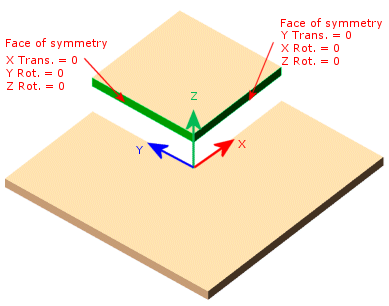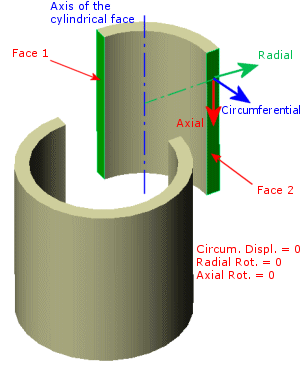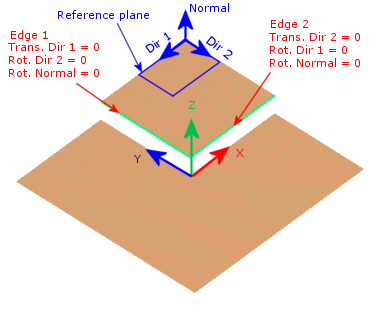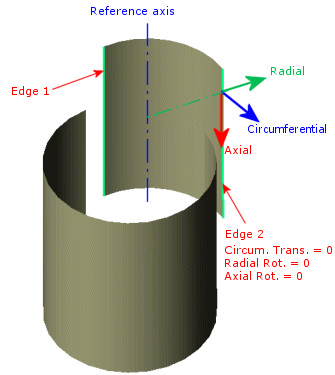# Symmetry Restraints for Shell Models

For shell models, symmetry requires that faces coinciding with planes of symmetry should be prevented from moving in the normal direction and rotating about the other two orthogonal directions. The planes of symmetry should be orthogonal to each other.

For sheet metals, symmetry restraints are applied on the faces coinciding with the planes of symmetry of the model along the model thickness. The plane of symmetry is always perpendicular to the shell surface where Symmetry is applied. The shell surface is mirrored about the plane of symmetry.

Use the Symmetry fixture under Advanced in the Fixture PropertyManager to apply a symmetry restraint to shell faces or shell edges. The shell edges must be planar. The shell faces must lie on orthogonal planes of symmetry.
Symmetry requires that geometry, restraints, loads, and material properties are symmetrical. In general, using symmetry is not recommended for buckling and frequency studies.

The following examples explain how symmetry restraints are applied to the different geometries:

## Sheet Metals that Mesh with Shell Elements

Planar SymmetryThis model is symmetric about two planes. You can analyze a quarter of the model. The Symmetry restraint automatically applies the translation and rotation conditions to the selected faces. The faces must be orthogonal to each other.

Axial SymmetryThis model is axisymmetric. You can analyze half or one quarter of the model. The Symmetry restraint automatically applies the translation and rotation conditions to the selected faces.

## Surfaces

Planar SymmetryThis surface model is symmetric about two planes. You can analyze a quarter of the model. Apply a Symmetry restraint to the shell edges. The selected edges must be orthogonal to each other.

The plane of symmetry is always perpendicular to the planar surface which the selected edge belongs. The shell surface is mirrored about the plane of symmetry.

Axial SymmetryThis surface model is axisymmetric. You can analyze half or one quarter of the model. Apply a Symmetry restraint to the shell edges.